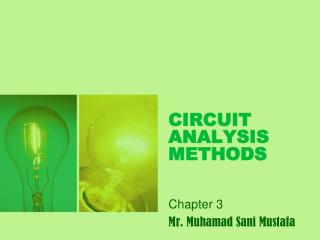DownloadDownload PresentationCIRCUIT ANALYSIS METHODS

# CIRCUIT ANALYSIS METHODS

Download Presentation## CIRCUIT ANALYSIS METHODS

- - - - - - - - - - - - - - - - - - - - - - - - - - - E N D - - - - - - - - - - - - - - - - - - - - - - - - - - -
##### Presentation Transcript

1. CIRCUIT ANALYSIS METHODS Chapter 3 Mr. Muhamad Sani Mustafa

2. CIRCUIT ANALYSIS METHODS • Node-Voltage method • Mesh-current method • Source transformation • Thevenin equivalent circuit • Norton equivalent circuit • Maximum power transfer • Superposition principle

3. INTRODUCTION OF NODE-VOLTAGE METHOD • Use KCL. • Important step: select one of the node as reference node • Then define the node voltage in the circuit diagram.

4. Node-voltage example

5. In the diagram, node 3 is define as reference node and node 1 and 2 as node voltage V1 and V2. • The node-voltage equation for node 1 is,

6. In the diagram, node 3 is define as reference node and node 1 and 2 as node voltage V1 and V2. • The node-voltage equation for node 1 is,

7. Node-voltage equation of node 2,

8. Solving for V1 and V2 yeilds

9. THE NODE-VOLTAGE METHOD AND DEPENDENT SOURCES • If the circuit contains dependent sources, the node-voltage equations must be supplemented with the constraint equation imposed by the presence of the dependent sources.

10. example… Use the node-voltage method to find the power dissipated in the 5Ω resistor.

11. The circuit has 3 node. • Thus there must be 2 node-voltage equation. • Summing the currents away from node 1 generates the equation,

12. Summing the current away from node 2 yields,

13. As written, these two equations contain three unknowns namely V1, V2 and iØ. • To eliminate iØ, express the current in terms of node-voltage,

14. Substituting this relationship into the node 2 equation,

15. Solving for V1 and V2 gives,

16. Then,

17. SPECIAL CASE • When a voltage source is the only element between two essential nodes, the node-voltage method is simplified.

18. Example…

19. There is three essential nodes, so two simultaneous equation are needed. • Only one unknown node voltage, V2 where as V1=100V. • Therefore, only a single node-voltage equation is needed which is at node 2.

20. Using V1 =100V, thus V2=125V.

21. SUPERNODE • When a voltage source is between two essential nodes, those nodes can be combine to form a supernode (voltage sourse is assume as open circuit).

22. Supernode example…

23. Nodes chosen,

24. Node-voltage equation for node 2 and 3,

25. Summing both equation, Above equation can be generates directly using supernode approach

26. Supernod

27. Starting with resistor 5Ω branch and moving counterclockwise around the supernode,

28. Using V1 =50V and V3 as a function of V2,

29. Substituded into the node-voltage equation,

30. Using V2 value, gives

31. CIRCUIT ANALYSIS METHODS • Node-Voltage method • Mesh-current method • Source transformation • Thevenin equivalent circuit • Norton equivalent circuit • Maximum power transfer • Superposition principle

32. INTRODUCTION OF MESH-CURRENT METHOD • A mesh is a loop with no loop inside it. • A mesh current is the current that exist only in the perimeter of a mesh. • Mesh-current method use KVL to generates equation for each mesh.

33. Mesh-current example…

34. Mesh-current circuit with mesh current ia and ib.

35. Use KVL on both mesh,

36. Solving for ia and ib, and you can compute any voltages or powers of interest.

37. THE MESH-CURRENT METHOD AND DEPENDENT SOURCES • If the circuit contains dependent sources, the mesh-current equations must be supplemented by the appropriate constraint equations.

38. Example…

39. Use the mesh-current method to determine the power dissipated in the 4Ω resistor.

40. Using KVL,

41. But • Substituting into the mesh-current equation,

42. Using Cramer rule, the values of i2 and i3 can be determine,

43. Power dissipated by 4Ω resistor is

44. SPECIAL CASE (SUPERMESH) • When a branch includes a current source, the mesh-current method can be simplified. • To create a supermesh, remove the current source from the circuit by simply avoiding the branch when writing the mesh-current equations.

45. Supermesh equation,